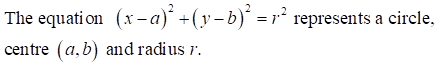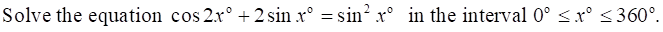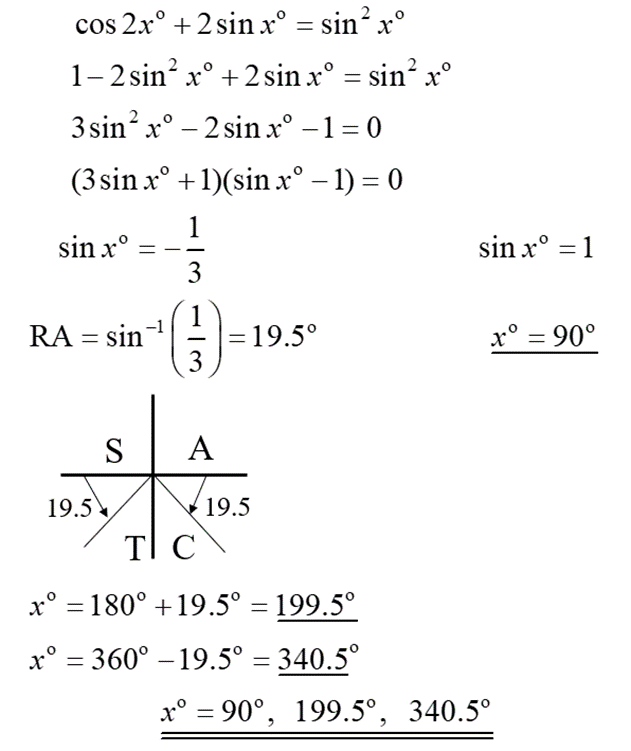top of page
Search

# Higher Maths Formula Sheet with notes and examplesThere's the Higher Maths Formula Sheet that you'll find in the SQA exam, and on some websites, and then there's our Higher Maths Formula Sheet! Ours has been specially made to provide extra support to Higher Maths students to explain which formulae you need to know and remember and which key areas from the course it is linked to. We've even provided examples from past paper questions where it applies, and of course, the solutions! This one is designed especially for the SQA 2022 Higher Maths exam to include only the areas which will be assessed.

Coming soon - SQA Higher Maths Past Papers

The Circle FormulaeIn exam questions often the equation of a circle is given in this form. You would use the information above to determine the centre and radius of the given circle.

The centre will be the coefficients of x and y divided by –2. Substitute these values and the constant c into the radius equation to find the radius.

Past Paper Example (Non-Calculator):Another equation of a circle:In exam questions when you are asked to find the equation of a circle, this is the formula that you should use. You need to find the centre and radius then substitute.

Past Paper Example (Non-Calculator):Trigonometric FormulaeNote that when using cos, if you have a ‘plus’ the expansion will have a ‘take away’.

Common exam questions using these formulae often involve calculating trig. ratios using SohCahToa. Then substituting these values into one of the above expansions.

Past Paper Example (Non-Calculator):Double Angle FormulaeThese formulae can be use in examples similar to the one above.

Very often you will use these formulae when solving trig. equations.

If it is sin2A that you have to replace, then you have no choice.

However, if it is cos2A you have to replace, then you have a choice to make.

From the 3 choices for cos2A when solving a trig. equation it will never be the first one.

If the other terms are cos then you should use:If the other terms are sin then you should use:Past Paper Example:Differentiation of sina and cosaThese formulae remind you that when you differentiate sin you get cos

and when you differentiate cos you get –sin.

The formulae given above also include the chain rule.

Past Paper Example (Non-Calculator):If you need support in National 5 or Higher subjects you can book classes here.